I.1.7b

12/22/20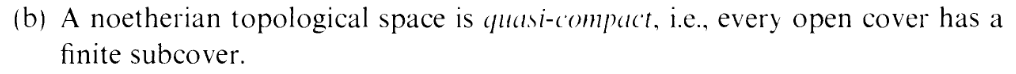Phew, an easy peasy exercise (or part of an exercise). Let's finish this baby off.

Let X be Noetherian, and suppose we're given an open cover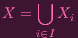(1)

First, pick any V 1 = Xi from the cover.

And now, more generally, suppose we have picked V 1,,V n from the cover. Let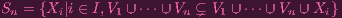(2)

Then pick any Xi Sn and let V n+1 = Xi

Now, if Sn was always nonempty, we can form a chain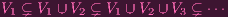(3)

(by the axiom of dependent choice, which I freaked out about in the last post) which would contradict Noetheriannness of X (see 1.7a). Hence Sn = for some n.

i.e.

 ∀i ∈ I: V 1 ∪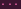∪ V n = V 1 ∪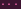∪ V n ∪ XiV 1 ∪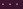∪ V n = ⋃ i∈IV 1 ∪∪ V n ∪ Xi = V 1 ∪∪ V n ∪⋃ i∈I Xi = V 1 ∪∪ V n ∪ X = X

So X = V 1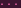V n is a finite subcover. Done.